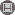Volume 14, Issue 1 (4-2019)                   IJMSI 2019, 14(1): 135-145 | Back to browse issues page

BibTeX | RIS | EndNote | Medlars | ProCite | Reference Manager | RefWorks
Send citation to:Mousavi H, Mohammadi R, Rahimi S. On Skew Cyclic Codes over a Finite Ring. IJMSI. 2019; 14 (1) :135-145
URL: http://ijmsi.ir/article-1-954-en.html
Abstract:

In this paper, we classify the skew cyclic codes over Fp +
vF_p + v^2F_p, where p is a prime number and v^3 = v. Each skew cyclic
code is a F_p+vF_p+v^2F_p-submodule of the (F_p+vF_p+v^2F_p)[x;alpha], where
v^3 = v and alpha(v) = -v. Also, we give an explicit forms for the generator of
these codes. Moreover, an algorithm of encoding and decoding for these
codes is presented.

Type of Study: Research paper | Subject: Special